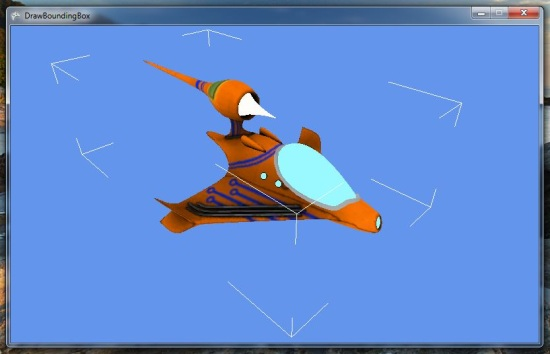# Drawing an XNA Model bounding box

• The source code for a working version is here.

If you’re making a level editor, or perhaps a model viewer, it can sometimes be useful to see a visualisation of the 3D space a model sits within. It might also be handy if you use bounding boxes for physics, and you want to check that the bounding box is really where you think it is.

## Creating the bounding box

First (and hopefully not surprisingly), you’ll need the bounding box itself. XNA has a nice structure for this, helpfully named BoundingBox. If you already have access to one of these for your model, then you can skip to the next section. If you only have a Model object, and want to creating a bounding box, then you can use this code. Note that the ModelMesh class has a BoundingSphere property. XNA allows us to create a BoundingBox from a BoundingSphere, but that wouldn’t be a tight fit - it would be larger than necessary. So I prefer to go back to the original vertices and use them to create the bounding box. Step 1, then, is to extract the vertex positions from the Model:

public static class VertexElementExtractor
{
public static Vector3[] GetVertexElement(ModelMeshPart meshPart, VertexElementUsage usage)
{
VertexDeclaration vd = meshPart.VertexBuffer.VertexDeclaration;
VertexElement[] elements = vd.GetVertexElements();

Func<VertexElement, bool> elementPredicate = ve => ve.VertexElementUsage == usage && ve.VertexElementFormat == VertexElementFormat.Vector3;
if (!elements.Any(elementPredicate))
return null;

VertexElement element = elements.First(elementPredicate);

Vector3[] vertexData = new Vector3[meshPart.NumVertices];
meshPart.VertexBuffer.GetData((meshPart.VertexOffset * vd.VertexStride) + element.Offset,
vertexData, 0, vertexData.Length, vd.VertexStride);

return vertexData;
}
}


We can now write the code to create a bounding box for a ModelMeshPart:

private static BoundingBox? GetBoundingBox(ModelMeshPart meshPart, Matrix transform)
{
if (meshPart.VertexBuffer == null)
return null;

Vector3[] positions = VertexElementExtractor.GetVertexElement(meshPart, VertexElementUsage.Position);
if (positions == null)
return null;

Vector3[] transformedPositions = new Vector3[positions.Length];
Vector3.Transform(positions, ref transform, transformedPositions);

return BoundingBox.CreateFromPoints(transformedPositions);
}


Now we can loop through each ModelMesh and ModelMeshPart within the Model, and create the merged bounding box for the whole model (making sure to transform the positions based on the bone transforms):

private static BoundingBox CreateBoundingBox(Model model)
{
Matrix[] boneTransforms = new Matrix[model.Bones.Count];
model.CopyAbsoluteBoneTransformsTo(boneTransforms);

BoundingBox result = new BoundingBox();
foreach (ModelMesh mesh in model.Meshes)
foreach (ModelMeshPart meshPart in mesh.MeshParts)
{
BoundingBox? meshPartBoundingBox = GetBoundingBox(meshPart, boneTransforms[mesh.ParentBone.Index]);
if (meshPartBoundingBox != null)
result = BoundingBox.CreateMerged(result, meshPartBoundingBox.Value);
}
return result;
}


In XBuilder, I actually create a bounding box for each ModelMesh, but the general principle is the same.

We now have a BoundingBox for our Model, so we can get ready to draw it.

## Preparing to draw the bounding box

You could draw an actual box, with solid lines. I prefer the approach taken by most 3D modelling packages of just drawing the corners. This is a bit more involved, and sorry for the long code snippet. If anybody knows a cleverer way of doing this, please let me know! First we need a class to hold the vertex and index buffers we’re going to create:

public class BoundingBoxBuffers
{
public VertexBuffer Vertices;
public int VertexCount;
public IndexBuffer Indices;
public int PrimitiveCount;
}


Now we can build this object:

private BoundingBoxBuffers CreateBoundingBoxBuffers(BoundingBox boundingBox, GraphicsDevice graphicsDevice)
{
BoundingBoxBuffers boundingBoxBuffers = new BoundingBoxBuffers();

boundingBoxBuffers.PrimitiveCount = 24;
boundingBoxBuffers.VertexCount = 48;

VertexBuffer vertexBuffer = new VertexBuffer(graphicsDevice,
typeof(VertexPositionColor), boundingBoxBuffers.VertexCount,
BufferUsage.WriteOnly);
List<VertexPositionColor> vertices = new List<VertexPositionColor>();

const float ratio = 5.0f;

Vector3 xOffset = new Vector3((boundingBox.Max.X - boundingBox.Min.X) / ratio, 0, 0);
Vector3 yOffset = new Vector3(0, (boundingBox.Max.Y - boundingBox.Min.Y) / ratio, 0);
Vector3 zOffset = new Vector3(0, 0, (boundingBox.Max.Z - boundingBox.Min.Z) / ratio);
Vector3[] corners = boundingBox.GetCorners();

// Corner 1.

// Corner 2.

// Corner 3.

// Corner 4.

// Corner 5.

// Corner 6.

// Corner 7.

// Corner 8.

vertexBuffer.SetData(vertices.ToArray());
boundingBoxBuffers.Vertices = vertexBuffer;

IndexBuffer indexBuffer = new IndexBuffer(graphicsDevice, IndexElementSize.SixteenBits, boundingBoxBuffers.VertexCount,
BufferUsage.WriteOnly);
indexBuffer.SetData(Enumerable.Range(0, boundingBoxBuffers.VertexCount).Select(i => (short)i).ToArray());
boundingBoxBuffers.Indices = indexBuffer;

return boundingBoxBuffers;
}

private static void AddVertex(List<VertexPositionColor> vertices, Vector3 position)
{
}


## Drawing the bounding box

By now you should have an instance of BoundingBoxBuffers. To get it on to the screen, you’ll need an Effect - I keep it simple and use BasicEffect:

BasicEffect lineEffect = new BasicEffect(graphicsDevice);
lineEffect.LightingEnabled = false;
lineEffect.TextureEnabled = false;
lineEffect.VertexColorEnabled = true;


Finally, we’re ready to draw the bounding box:

private void DrawBoundingBox(BoundingBoxBuffers buffers, BasicEffect effect, GraphicsDevice graphicsDevice, Matrix view, Matrix projection)
{
graphicsDevice.SetVertexBuffer(buffers.Vertices);
graphicsDevice.Indices = buffers.Indices;

effect.World = Matrix.Identity;
effect.View = view;
effect.Projection = projection;

foreach (EffectPass pass in effect.CurrentTechnique.Passes)
{
pass.Apply();
graphicsDevice.DrawIndexedPrimitives(PrimitiveType.LineList, 0, 0,
buffers.VertexCount, 0, buffers.PrimitiveCount);
}
}


If everything went right, you should have something that looks like this on your screen:If you don’t have that, or if I didn’t explain it properly, maybe looking at my version will help. I hope this is useful - let me know if you have any comments or questions.